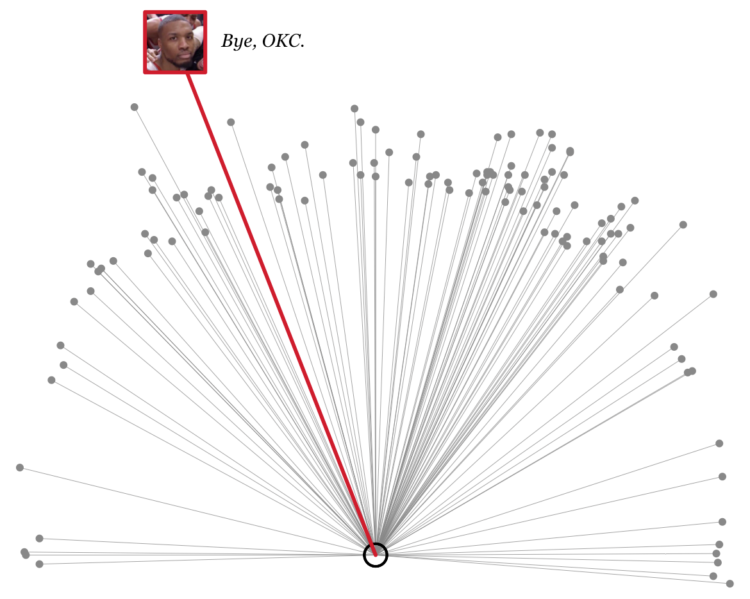# Damian Lillard’s Game-Winner in Context

Damian Lillard of the Portland Trailblazers hit a crazy game-winner the other night. The game was tied, the clock was winding down, and Lillard pulled up from a thousand feet away for the win. Lillard’s straight-faced reaction was as good as the shot.

Here’s how that shot matches up with all of the other threes Lillard has made during his playoff career:Just nuts.

The R code, in case you’re interested:

```library(png)
library(plotrix)

par(mar=c(0,0,0,0))
plot(-makes3\$loc_x[-dim(makes3)], makes3\$loc_y[-dim(makes3)],
cex=.7, pch=19, col="#888888",
asp=1, bty="n", axes=FALSE, xlab="", ylab="",
xlim=c(-25, 25), ylim=c(0, 50))
segments(-makes3\$loc_x[-dim(makes3)], makes3\$loc_y[-dim(makes3)],
rep(0, dim(makes3)), rep(5.25, dim(makes3)),
lwd=.4, col="#888888")
draw.arc(0, 5.25, 9/12, angle1=0, angle2=2*pi, col="black", lwd=2)

# Game winner
x_win <- -makes3\$loc_x[dim(makes3)]
y_win <- makes3\$loc_y[dim(makes3)]
segments(x_win, y_win, 0, 5.25, lwd=3, col="#CF082C")